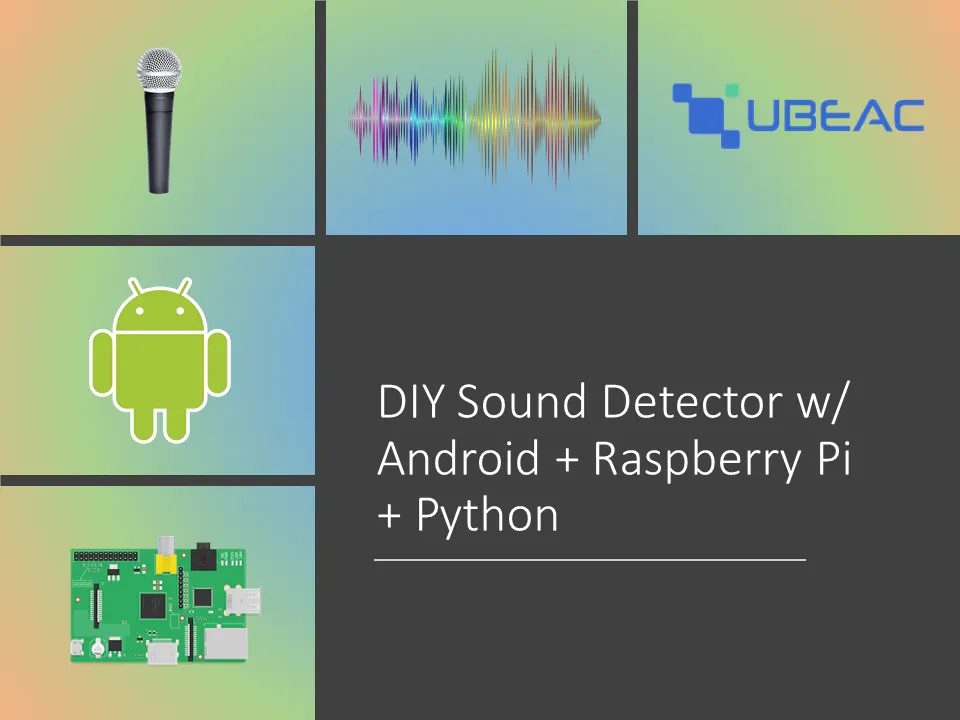# DIY Sound Detector w/ Android + Raspberry Pi

Hide a microphone in plain sight using an old Android device. Stream the audio to a Raspberry Pi and visualize it in an IoT Dashboard!

IntermediateFull instructions provided2 hours499## Things used in this project

### Hardware componentsAndroid device
×1Raspberry Pi 3 Model B Optional
×1

### Software apps and online servicesuBeac IoT Platform Free Subscription

## Code

### Sound Detector

Python
```import json
import http.client
from _util import get_sensor
import urllib.request
import math
import scipy.io.wavfile
from scipy.fftpack import fft, fftfreq
import numpy as np
import struct

framerate = 8000
time_streaming = 5 #seconds
stream_length = time_streaming * 4
nframes = int(stream_length * framerate / 4)
file_location = "INSERT FILE LOCATION HERE"

# Configuration section
UBEAC_URL = 'hub.ubeac.io'
GATEWAY_URL = 'INSERT GATEWAY URL HERE'
DEVICE_FRIENDLY_NAME = 'Android Sound Detector'

with urllib.request.urlopen('INSERT LANMIC URL HERE') as r:
while True:
for i in range(stream_length - 1):

format_float = '<' + str(nframes) + 'i'

nb = np.array(testResult)
nm = np.max(np.abs(nb))
sigf32 = (nb/nm).astype(np.float32)
scipy.io.wavfile.write(file_location, framerate, sigf32)

rms_amp = np.sqrt(np.mean(np.square(data)))
logrms_amp = 20 * math.log10(rms_amp)

Amplitude = get_sensor("Average Amplitude", {"Amplitude": str(logrms_amp)})

freqs = fftfreq(data.shape, 1/rate)
freqspos = freqs[:int(freqs.size/2)]
datafft = fft(data)
fftabs = abs(datafft)[:int(freqs.size/2)]

peakfreq = np.max(fftabs)
locmaxfreq = np.argmax(fftabs)
freqmax = freqspos[locmaxfreq]

Frequency = get_sensor("Frequency", {"Max Frequency" : str(freqmax)})

Peak = get_sensor("Max Peak", {"Amplitude" : str(locmaxfreq)})

sensors = []
sensors.append(Amplitude)
sensors.append(Frequency)
sensors.append(Peak)

device = [{
'id': "Android Microphone",
'sensors': sensors
}]

connection = http.client.HTTPSConnection(UBEAC_URL)
connection.request('POST', GATEWAY_URL, json.dumps(device))
response = connection.getresponse()
```

### _util.py

Python
```def to_mega_byte(byte_value):
return int(byte_value / 1048576)

def to_giga_byte(byte_value):
return int(byte_value / 173741824)

def get_sensor(id, value, type=None, unit=None, prefix=None, dt=None):
sensor = {
'id': id,
'data': value
}
return sensor

def secs2hours(secs):
return round(secs / 3600, 1)
```

## Credits

### Amir Pournasserian

7 projects • 3 followers
Data scientist, machine learning specialist, IoT nerd. CEO of Momentaj and Founder of uBeac, IoT thinktanks.